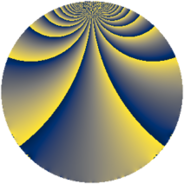# Properties

 Label 231.2.wLevel $231$ Weight $2$ Character orbit 231.w Rep. character $\chi_{231}(13,\cdot)$ Character field $\Q(\zeta_{10})$ Dimension $64$ Newform subspaces $1$ Sturm bound $64$ Trace bound $0$

# Related objects

## Defining parameters

 Level: $$N$$ $$=$$ $$231 = 3 \cdot 7 \cdot 11$$ Weight: $$k$$ $$=$$ $$2$$ Character orbit: $$[\chi]$$ $$=$$ 231.w (of order $$10$$ and degree $$4$$) Character conductor: $$\operatorname{cond}(\chi)$$ $$=$$ $$77$$ Character field: $$\Q(\zeta_{10})$$ Newform subspaces: $$1$$ Sturm bound: $$64$$ Trace bound: $$0$$

## Dimensions

The following table gives the dimensions of various subspaces of $$M_{2}(231, [\chi])$$.

Total New Old
Modular forms 144 64 80
Cusp forms 112 64 48
Eisenstein series 32 0 32

## Trace form

 $$64q + 12q^{4} + 10q^{7} - 20q^{8} + 16q^{9} + O(q^{10})$$ $$64q + 12q^{4} + 10q^{7} - 20q^{8} + 16q^{9} - 16q^{11} + 12q^{14} - 12q^{15} - 16q^{16} + 10q^{18} - 40q^{22} - 24q^{23} + 44q^{25} - 30q^{28} - 40q^{29} - 40q^{35} - 12q^{36} + 32q^{37} - 2q^{42} + 22q^{44} - 70q^{46} - 50q^{49} - 40q^{51} - 64q^{53} + 80q^{56} + 2q^{58} - 36q^{60} + 10q^{63} + 72q^{64} - 8q^{67} - 26q^{70} + 68q^{71} - 10q^{72} + 80q^{74} + 90q^{77} - 72q^{78} + 40q^{79} - 16q^{81} + 60q^{84} - 40q^{85} - 62q^{86} + 140q^{88} + 54q^{91} + 18q^{92} - 20q^{93} + 20q^{95} - 4q^{99} + O(q^{100})$$

## Decomposition of $$S_{2}^{\mathrm{new}}(231, [\chi])$$ into newform subspaces

Label Dim. $$A$$ Field CM Traces $q$-expansion
$$a_2$$ $$a_3$$ $$a_5$$ $$a_7$$
231.2.w.a $$64$$ $$1.845$$ None $$0$$ $$0$$ $$0$$ $$10$$

## Decomposition of $$S_{2}^{\mathrm{old}}(231, [\chi])$$ into lower level spaces

$$S_{2}^{\mathrm{old}}(231, [\chi]) \cong$$ $$S_{2}^{\mathrm{new}}(77, [\chi])$$$$^{\oplus 2}$$